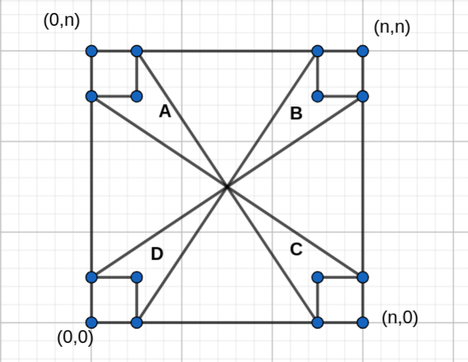# Arealiar_rakibLong Practice Sessions on...
Limits 1s, 512 MB

In this problem, you will be given a square which has a length of n. Co-ordinates of the square are (0,0), (n,0),(n,n),(0,n) .
You need to draw 4 straight lines:

1. Line from (0,1) to (n,n-1)
2. Line from (1,0) to (n-1,n)
3. Line from (0,n-1) to (n,1)
4. Line from (1,n) to (n-1,0)

These four lines will intersect in a point (x,y) like the figure shown below.

Calculate the total area of A+B+C+D (except the four corner unit square).## Input

Input will start with an integer T. Then there will be T cases. Each case will contain one integer N.
1 <= T <= 100000
3 <= n <= 1018

## Output

For each test case, print "Case x: y" without quotation marks where x is the case number and y is the required answer.

It is guaranteed that y is always an integer.

## Sample

InputOutput
1
6
Case 1: 8### Submit